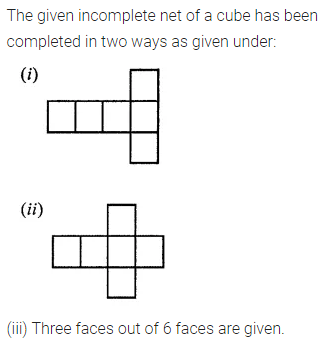# ML Aggarwal Class 7 Solutions for ICSE Maths Chapter 15 Visualising Solid Shapes Ex 15.1

## ML Aggarwal Class 7 Solutions for ICSE Maths Chapter 15 Visualising Solid Shapes Ex 15.1

Question 1.
Match the following shapes with their names:Solution: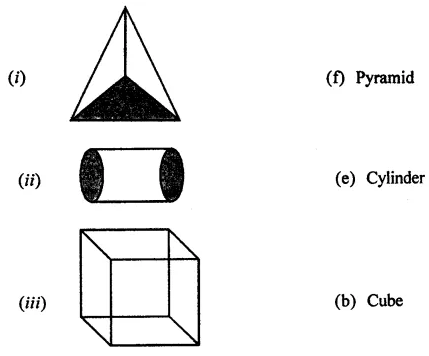Question 2.
Identify the nets which can be folded to form a cube (cut out copies of the nets and try it):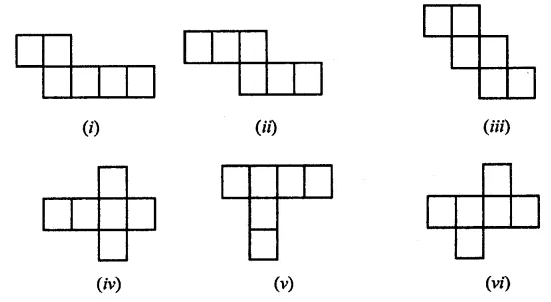Solution:Question 3.
Dice are cubes with dots on each face. Opposite faces of a die always have a total of seven dots on them.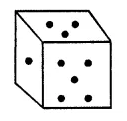Here are two nets to make dice (cubes); the numbers inserted in each square indicate the number of dots in that box.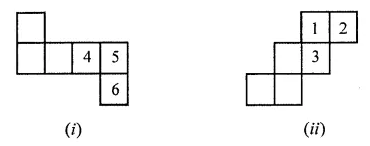Insert suitable numbers in the blanks, remembering that the number on the opposite faces should total to 7.
Solution: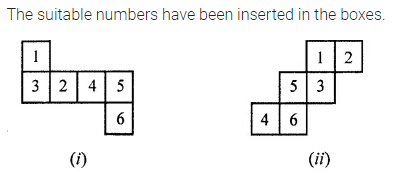Question 4.
Can any of the following be a net for a die? If no, explain your answer: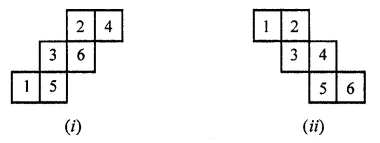Solution:Question 5.
Here is an incomplete net for making a cube. Complete it in at least two different ways. Remember that a cube has six faces. How many are there in the net here? (You may use a squared paper for easy manipulation.)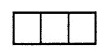Solution: